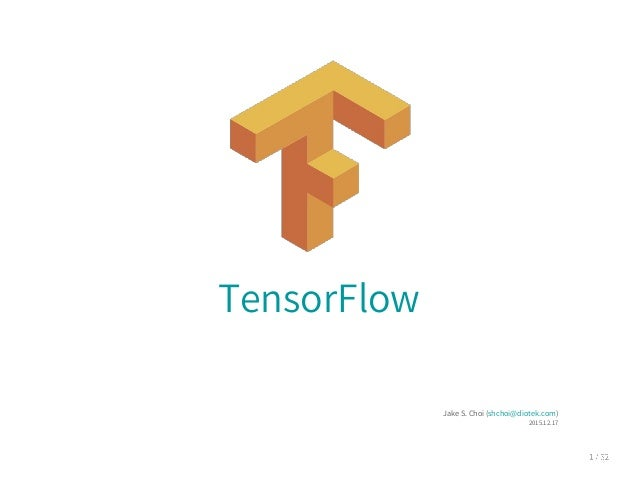Successfully reported this slideshow.
We use your LinkedIn profile and activity data to personalize ads and to show you more relevant ads. You can change your ad preferences anytime.Upcoming SlideShare
×

# TensorFlow

6,537 views

Published on

TensorFlow에 대한 분석 내용

- TensorFlow?
- 배경
- DistBelief
- Tutorial - Logistic regression
- TensorFlow - 내부적으로는
- Tutorial - CNN, RNN
- Benchmarks
- 다른 오픈 소스들
- TensorFlow를 고려한다면
- 설치
- 참고 자료

Published in: Engineering
• Full Name
Comment goes here.

Are you sure you want to Yes No
Your message goes here• Be the first to comment

### TensorFlow

1. 1. TensorFlow JakeS.Choi(shchoi@diotek.com) 2015.12.17
2. 2. 차례 TensorFlow? 배경 DistBelief Tutorial-Logisticregression TensorFlow-내부적으로는 Tutorial-CNN,RNN Benchmarks 다른오픈소스들 TensorFlow를고려한다면 설치 참고자료
3. 3. TensorFlow? Opensourcesoftwarelibraryfornumericalcomputation usingdataflowgraphs Deeplearningframework이아니네? GoogleResearchBlog에2015년11월9일발표 Apache2.0라이선스
4. 4. Tensor-Flow? Large-ScaleDeepLearningforIntelligentComputerSystems
5. 5. 배경 2012년기준으로구글내부적으로machinelearning,deep learning에대한수요가증가함 Large-ScaleDeepLearningforIntelligentComputerSystems GMail,Photos,Speech등어러분야에적용
6. 6. 배경 2011년GoogleBrainproject의일환으로대용량데이터와대규모계 산을중점으로개발을시작 Large-ScaleDeepLearningforIntelligentComputerSystems
7. 7. DistBelief 2011년에개발된Google의Deeplearninginfrastructure 기본설계방향 처리할데이터가많다→데이터병렬화 모델이너무커서메모리에한번에안올라감→모델병렬화 구글은학습에천단위코어수를사용함 Introductiontoapachehorn(incubating)
8. 8. DistBelief Modelparallelismbenchmarks 실험결과 HWR은일반적으로40K,최대10M까지테스트 Speech의경우8대에서2.2배의성능향상,8대이상은데이터교환 비용으로성능하락이확인됨 LargeScaleDistributedDeepNetworks
9. 9. DistBelief Dataparallelismbenchmarks 실험결과 LargeScaleDistributedDeepNetworks
10. 10. TensorFlow 기존DistBelief를개선 DistBelief은규모에대한확장성은뛰어나지만유연성이떨어졌음 TensorFlow는DistBelief보다는2배정도빠름다고함 아직whitepaper에benchmarking자료는미포함됨 구글내부적으로는DistBelief에서TensorFlow로이전을완료한상태
11. 11. Tutorial-Logisticregression 0~9숫자하나를인식하는문제,문제의해결을위한모델은logistic regression을사용 MNIST파일을로딩,input_data는구글에서미리구현해둔코드 #ImportMINSTdata importinput_data mnist=input_data.read_data_sets(MNIST_data/,one_hot=True) 데이터는아래와같은형태의이미지 MNISTForMLBeginners
12. 12. Tutorial-Logisticregression mnist.train,mnist.validation,mnist.test로구성 mnist.*.xs는아래와같은형태의28x28x1(Grayscale)이미지 MNISTForMLBeginners
13. 13. Tutorial-Logisticregression 총60,000의이미지로구성 MNISTForMLBeginners
14. 14. Tutorial-Logisticregression mnist.*.ys는0~9까지하나만1을가지는one-hotvector로목표/정 답데이터 MNISTForMLBeginners
15. 15. Tutorial-Logisticregression importtensorflowastf #Parameters learning_rate=0.01 training_epochs=25 batch_size=100 display_step=1 #tfGraphInput x=tf.placeholder(float,[None,784])#mnistdataimageofshape28*28=784 y=tf.placeholder(float,[None,10])#0-9digitsrecognition=10classes #Createmodel #Setmodelweights W=tf.Variable(tf.zeros([784,10])) b=tf.Variable(tf.zeros()) #Constructmodel activation=tf.nn.softmax(tf.matmul(x,W)+b)#Softmax #Minimizeerrorusingcrossentropy cost=-tf.reduce_sum(y*tf.log(activation))#Crossentropy #GradientDescent optimizer=tf.train.GradientDescentOptimizer(learning_rate).minimize(cost)
16. 16. Tutorial-Logisticregression Nonex784(28x28)으로x라는matrix를정의,placeholder로정의된 내용은그래프계산전전달되어야함,이값은외부에서그래프로입력을 다룰때사용y는정답데이터가입력됨,여기서None으로정의된값은 mini-batchsize가됨 x=tf.placeholder(float,[None,784]) y=tf.placeholder(float,[None,10])#0-9digitsrecognition=10classes W는inputxoutput크기의matrix,b는bias로output크기와동일한 변수를정의 W=tf.Variable(tf.zeros([784,10])) b=tf.Variable(tf.zeros())
17. 17. Tutorial-Logisticregression logisticregression모델을정의 tf.matmul은matrixmultiply함수 activation=tf.nn.softmax(tf.matmul(x,W)+b)#Softmax
18. 18. Tutorial-Logisticregression crossentropy함수 cost함수를위의수식과같이crossentropy함수와동일하게정의 optimizier는아래와같이gradientdecent를사용하고cost가최소화 되도록최적화를실행 cost=-tf.reduce_sum(y*tf.log(activation))#Crossentropy #GradientDescent optimizer=tf.train.GradientDescentOptimizer(learning_rate).minimize(cost)
19. 19. Tutorial-Logisticregression #Initializingthevariables init=tf.initialize_all_variables() #Launchthegraph withtf.Session()assess: sess.run(init) #Trainingcycle forepochinrange(training_epochs): avg_cost=0. total_batch=int(mnist.train.num_examples/batch_size) #Loopoverallbatches foriinrange(total_batch): batch_xs,batch_ys=mnist.train.next_batch(batch_size) #Fittrainingusingbatchdata sess.run(optimizer,feed_dict={x:batch_xs,y:batch_ys}) #Computeaverageloss avg_cost+=sess.run(cost,feed_dict={x:batch_xs,y:batch_ys})/total_batch #Displaylogsperepochstep ifepoch%display_step==0: printEpoch:,'%04d'%(epoch+1),cost=,{:.9f}.format(avg_cost)
20. 20. Tutorial-Logisticregression #Initializingthevariables init=tf.initialize_all_variables() #Launchthegraph withtf.Session()assess: ... printOptimizationFinished! #Testmodel correct_prediction=tf.equal(tf.argmax(activation,1),tf.argmax(y,1)) #Calculateaccuracy accuracy=tf.reduce_mean(tf.cast(correct_prediction,float)) printAccuracy:,accuracy.eval({x:mnist.test.images,y:mnist.test.labels})
21. 21. Tutorial-Logisticregression 코드를실행하면아래와같이dataflowgraph를생성함 Large-ScaleDeepLearningforIntelligentComputerSystems
22. 22. Tutorial-Logisticregression 이렇게만들어진그래프는분산처리가가능함 Large-ScaleDeepLearningforIntelligentComputerSystems
23. 23. Tutorial-Logisticregression 코드상에는forwardpass만있고backwardpass에대한내용이없 음(Optimizer정의를제외하고) TensorFlow에서는x,y,W,b등을각각의심볼로다루고심볼계산 (Symboliccomputation)을지원함 내부적으로자동적으로미분함수를구하고gradient를계산함
24. 24. Tutorial-Logisticregression GraphVisualization 아래와같이summary에관한코드를추가 #Initializingthevariables init=tf.initialize_all_variables() tf.scalar_summary(loss,cost) merged_summary_op=tf.merge_all_summaries() #Launchthegraph withtf.Session()assess: sess.run(init) summary_writer=tf.train.SummaryWriter('/tmp/tf_l_regression.log',graph_def= sess.graph_def) ...
25. 25. Tutorial-Logisticregression GraphVisualization ... #Trainingcycle forepochinrange(training_epochs): avg_cost=0. total_batch=int(mnist.train.num_examples/batch_size) #Loopoverallbatches foriinrange(total_batch): batch_xs,batch_ys=mnist.train.next_batch(batch_size) #Fittrainingusingbatchdata sess.run(optimizer,feed_dict={x:batch_xs,y:batch_ys}) #Computeaverageloss avg_cost+=sess.run(cost,feed_dict={x:batch_xs,y:batch_ys})/total_batch summary_str=sess.run(merged_summary_op,feed_dict={x:batch_xs,y:bat ch_ys}) summary_writer.add_summary(summary_str,epoch*total_batch+i) ...
26. 26. Tutorial-Logisticregression GraphVisualization Tensorflow가동작중로그를지정한경로에남김 그런후아래와같이실행하면http://localhost:6006에접속이가능함 tensorboard--logdir=/tmp/tf_l_regression.log
27. 27. Tutorial-Logisticregression GraphVisualization 아래와같이남겨진scalar값은그림과같이값의변화를확인할수있음 tf.scalar_summary(loss,cost)
28. 28. Tutorial-Logisticregression GraphVisualization
29. 29. TensorFlow-돌아와서 DNN뿐아니라다른machinelearing등대규모matrix연산이필요 한곳에적용이가능함 하드웨어에대한이해가필요없음 Deeplearning을위한다양한최적화된함수를지원 자동미분을계산하여backwardpass에대한코드작성이불필요함 다양한모델과결합이쉬움 C++,Pythonfrontend를지원 Large-ScaleDeepLearningforIntelligentComputerSystems
30. 30. TensorFlow-내부적으로는 분산,이종/다중디바이스를위한고려,현재는singlemachine코드만 공개된상태 구글에서도해당기능에대해서중요하게생각하고있으며향후공개가 능성이높을것으로판단됨 Multi-device(GPU/CPU)에대한동작은지원하고있음 TensorFlow:Large-scalemachinelearningonheterogeneoussystems
31. 31. TensorFlow-내부적으로는 Scheduling과리소스(CPU,GPU,메모리등)별node배치가핵심일 것으로판단됨 노드배치는costmodel을사용함 Cost는input,output의크기와operation으로통계적으로추정 혹은이미실행된배치에서측정된비용으로계산됨 이비용에는계산,데이터교환비용등이포함됨 사용자에의해특정작업을특정디바이스에할당할수도있음 디바이스간간선은동적으로send/receivenode가추가되어디바이 스로부터추상화시킴 TensorFlow:Large-scalemachinelearningonheterogeneoussystems
32. 32. TensorFlow-내부적으로는 특별히Gradient기반의그래프계산에는몇가지힌트를사용할수있 음 forwardpass를통해계산된결과는backwardpass에서다시사 용됨 따라서노드할당에이를고려하여할당 단,메모리한계가있기때문에long-livedtensor에대한 swapping도같이고려됨 TensorFlow:Large-scalemachinelearningonheterogeneoussystems
33. 33. TensorFlow-내부적으로는 커널구현은cuBLAS,cuDNN,Eigen등을사용 데이터병렬화에대한내용에대한고려가되어있음 그래프생성후디바이스별노드배치는모델병렬화의내용 아직이부분도API상에서확인되지않음 TensorFlow:Large-scalemachinelearningonheterogeneoussystems
34. 34. Tutorial-CNN importtensorflowastf importinput_data defweight_variable(shape): #mean=0.0 initial=tf.truncated_normal(shape,stddev=0.1) returntf.Variable(initial) defbias_variable(shape): initial=tf.constant(0.1,shape=shape) returntf.Variable(initial) defconv2d(x,W): returntf.nn.conv2d(x,W,strides=[1,1,1,1],padding='SAME') defmax_pool_2x2(x): returntf.nn.max_pool(x,ksize=[1,2,2,1],strides=[1,2,2,1],padding='SAME')
35. 35. Tutorial-CNN mnist=input_data.read_data_sets('MNIST_data/',one_hot=True) x=tf.placeholder('float',[None,784]) #-1==batchsize x_image=tf.reshape(x,[-1,28,28,1]) #5x5patchsizeof1channel,32features W_conv1=weight_variable([5,5,1,32]) b_conv1=bias_variable() h_conv1=tf.nn.relu(conv2d(x_image,W_conv1)+b_conv1) h_pool1=max_pool_2x2(h_conv1) #5x5patchsizeof32channel,64features W_conv2=weight_variable([5,5,32,64]) b_conv2=bias_variable() h_conv2=tf.nn.relu(conv2d(h_pool1,W_conv2)+b_conv2) h_pool2=max_pool_2x2(h_conv2)
36. 36. Tutorial-CNN #imagesizereducedto7x7,fullconnected W_fc1=weight_variable([7*7*64,1024]) b_fc1=bias_variable() h_pool2_flat=tf.reshape(h_pool2,[-1,7*7*64]) h_fc1=tf.nn.relu(tf.matmul(h_pool2_flat,W_fc1)+b_fc1) keep_prob=tf.placeholder('float') h_fc1_drop=tf.nn.dropout(h_fc1,keep_prob) W_fc2=weight_variable([1024,10]) b_fc2=bias_variable() y_conv=tf.nn.softmax(tf.matmul(h_fc1_drop,W_fc2)+b_fc2) y_=tf.placeholder('float',[None,10]) cross_entropy=-tf.reduce_sum(y_*tf.log(y_conv))
37. 37. Tutorial-CNN train_step=tf.train.AdamOptimizer(1e-4).minimize(cross_entropy) correct_prediction=tf.equal(tf.argmax(y_conv,1),tf.argmax(y_,1)) accuracy=tf.reduce_mean(tf.cast(correct_prediction,'float'))
38. 38. Tutorial-RNN JürgenSchmidhuber가제안한문제 timestep 0 1 2 3 4 ... 20 21 ... 53 54 x 0.5 0.7 0.3 0.1 0.2 ... 0.5 0.9 ... 0.8 0.2 x 0 0 1 0 0 0 1 0 0 이입력은0.3+.9=1.2가정답으로출력되어야함 rnn_example.ipynb
39. 39. Tutorial-RNN 아래코드는이문제를생성하는코드 defgen_data(min_length=51,max_length=55,n_batch=5): X=np.concatenate([np.random.uniform(size=(n_batch,max_length,1)), np.zeros((n_batch,max_length,1))], axis=-1) y=np.zeros((n_batch,)) #Computemasksandcorrectvalues forninrange(n_batch): #Randomlychoosethesequencelength length=np.random.randint(min_length,max_length) #ichangedthistoaconstant #length=55 #ZerooutXaftertheendofthesequence X[n,length:,0]=0 #Settheseconddimensionto1attheindicestoadd X[n,np.random.randint(length/2-1),1]=1 X[n,np.random.randint(length/2,length),1]=1 #MultiplyandsumthedimensionsofXtogetthetargetvalue y[n]=np.sum(X[n,:,0]*X[n,:,1]) return(X,y)
40. 40. Tutorial-RNN importtensorflowastf importnumpyasnp fromtensorflow.models.rnnimportrnn_cell fromtensorflow.models.rnnimportrnn #Definingsomehyper-params num_units=2#thisistheparameterforinput_sizeinthebasicLSTMcell input_size=2#num_unitsandinput_sizewillbethesame batch_size=50 seq_len=55 num_epochs=100
41. 41. Tutorial-RNN ###ModelConstruction #weusethebasicLSTMcellprovidedinTensorFlow cell=rnn_cell.BasicLSTMCell(num_units) #numunitsistheinput-sizeforthiscell #createplaceholdersforXandy inputs=[tf.placeholder(tf.float32,shape=[batch_size,input_size])for_inrange(se q_len)] result=tf.placeholder(tf.float32,shape=[batch_size]) #notethatoutputsisalistofseq_len outputs,states=rnn.rnn(cell,inputs,dtype=tf.float32) #eachelementisatensorofsize[batch_size,num_units] #weactuallyonlyneedthelastoutputfromthemodel,ie:lastelementofoutputs
42. 42. Tutorial-RNN #Weactuallywanttheoutputtobesize[batch_size,1] #Sowewillimplementalinearlayertodothis W_o=tf.Variable(tf.random_normal([2,1],stddev=0.01)) b_o=tf.Variable(tf.random_normal(,stddev=0.01)) outputs2=outputs[-1] outputs3=tf.matmul(outputs2,W_o)+b_o #computethecostforthisbatchofdata cost=tf.reduce_mean(tf.pow(outputs3-result,2)) #computeupdatestoparametersinordertominimizecost train_op=tf.train.RMSPropOptimizer(0.005,0.2).minimize(cost)
43. 43. Tutorial-RNN controlflow중loopcontrol에대한지원이없어Pythonloop로동 작하여성능이슈가있을것으로판단됨 BLSTM,CTC은미구현,가변길이의RNN입력이불가
44. 44. Benchmarks convnet-benchmarks의자료 AlexNet-Input128x3x224x224 Library Class Time(ms) forward(ms) backward(ms) Nervana-fp16 ConvLayer 92 29 62 CuDNN[R3]-fp16 cudnn.SpatialConvolution 96 30 66 CuDNN[R3]-fp32 cudnn.SpatialConvolution 96 32 64 Nervana-fp32 ConvLayer 101 32 69 fbfft fbnn.SpatialConvolution 104 31 72 cudaconvnet2* ConvLayer 177 42 135 CuDNN[R2]* cudnn.SpatialConvolution 231 70 161 Caffe(native) ConvolutionLayer 324 121 203 TensorFlow conv2d 326 96 230 Torch-7(native) SpatialConvolutionMM 342 132 210 CL-nn(Torch) SpatialConvolutionMM 963 388 574 Caffe-CLGreenTea ConvolutionLayer 1442 210 123
45. 45. 다른오픈소스들 Theano 2009년부터시작 Symbolicgraph처리를TensorFlow보다먼저구현한라이브러리 scan이라불리는loopingcontrol을지원하여RNN구현에용이함 Block,Keras와같은Theano를기반으로한상위레벨의라이브러리 가존재함 Keras는backend로TensorFlow를지원하고있음 Multi-GPU에대한지원이없음 Python기반 Touch 2002년부터시작 RNN에대한지원이없음 비공식적브랜치에서는지원 YannLeCun이contributor로있는프로젝트 Lua기반 EvaluationofDeepLearningToolkits
46. 46. 다른오픈소스들 기타 Caffe:2013년UCBerkeley,Yangqingjia개발 Deeplearning4J:2014년AdamGibson이개발 DeepDist:2014년Facebook,Dirkneumann이개발 ApacheHorn:2012년분산ANN을기반한Hama에서파생됨, DistBelief오픈소스화를위한국내개발자프로젝트 EvaluationofDeepLearningToolkits
47. 47. 다른오픈소스들 Keras 지원하는기능이라면구조명세수준 fromkeras.modelsimportSequential fromkeras.layers.coreimportDense,Dropout,Activation fromkeras.layers.embeddingsimportEmbedding fromkeras.layers.recurrentimportLSTM model=Sequential() model.add(Embedding(max_features,256,input_length=maxlen)) model.add(LSTM(output_dim=128,activation='sigmoid',inner_activation='hard_si gmoid')) model.add(Dropout(0.5)) model.add(Dense(1)) model.add(Activation('sigmoid')) model.compile(loss='binary_crossentropy',optimizer='rmsprop') model.fit(X_train,Y_train,batch_size=16,nb_epoch=10) score=model.evaluate(X_test,Y_test,batch_size=16)
48. 48. TensorFlowv0.5 분산처리관련구현미공개 C++frontend를지원하나Python쪽에집중되어있음 C++에서는그래프생성,자동미분계산등이누락되어있음 C++예제코드는Python에서그래프생성하여파일(protocal buffer포맷)로저장후로딩하도록되어있음 그래프생성코드는Python으로작성된것으로추정됨 EEG라불리는성능프로파일링툴은미공개상태 TensorFlow:Large-scalemachinelearningonheterogeneoussystems
49. 49. TensorFlow를고려한다면 작은규모의파라미터에서도나쁘지않은성능 아직분산처리는공개되지않았지만단일GPU에서모델이다올라가지 않는다면대안을가진라이브러리는많지않음 RNN쪽지원은아직더기다려야할것같음 v0.5에비해나쁘지않는완성도 구느님의V8엔진같은신적화를기대해볼수있을지도 TensorFlow뿐아니라다른라이브러리도구현코드가줄어들어모델 자체에집중할수있을것으로보임 Keras같은high-level라이브러리를선택하여backend를변경해 도될것같음 이제WYSIWYG툴만나오면됨
50. 50. 설치 GPU요구사항 CUDA7.0,cuDNN6.5 CUDA(Computecapability)버전3.5이상,비공식적으로는 CUDA3.0GPU도지원 Titan,Tesla(K20,K40)권장 Multiprocessor최소8개이상 OS:Linux,OSX Python2.7 Windows는아직지원하지않음 DockerToolbox를통해시도는가능 버전이다른라이브러리는시도하지않는것이정신건강에좋음
51. 51. 설치 TensorFlow를동작하는방법은3가지방법이있음 Docker이미지 가장편안한방법 Docker사용법을익혀야함 Python패키지 로컬에서작업가능 소스컴파일이필요한작업은확인불가능,몇몇예제는bazel빌드로 동작 소스빌드 가장어려운방법 약간은bazel을익혀야함
52. 52. 설치-Docker tensorflow,tensorflow-full두가지이미지가있으며tensorflow-full 은소스가포함되어있음 dockerrun-p127.0.0.1:8888:8888-itb.gcr.io/tensorflow/tensorflow-full -p127.0.0.1:8888:8888는jupyter(iPython)포트를포워딩하기위함 아래와같이jupyter실행이가능함 root@bacf85f6aea3:~#/run_jupyter.sh
53. 53. 설치-Docker(GPU) Dockertensorflow,tensorflow-full이미지는GPU를지원하지않음 Docker내부에서GPU를동작하게하려면소스를받아야함 gitclone--recurse-submoduleshttps://github.com/tensorflow/tensorflow GPU사용시아래와같은환경변수가등록되어야함 exportLD_LIBRARY_PATH=\$LD_LIBRARY_PATH:/usr/local/cuda/lib64 exportCUDA_HOME=/usr/local/cuda tensorflow/tensorflow/tools/docker에docker_run_gpu.sh를실행 하면local의환경변수를읽어docker이미지를생성함
54. 54. 설치-Python Python2.7을사용함아직3.0은지원하지않음,만약여러버전이공존 한다면virtualenv사용을권장 python-pip,python-dev,python-virtualenv는설치되어있어야함,아 래예시는GPU지원pip패키지를설치 \$virtualenv--system-site-packages-p/usr/bin/python2.7tensorflow \$source~/tensorflow/bin/activate (tensorflow)\$pipinstall--upgradehttps://storage.googleapis.com/tensorflow/linux /gpu/tensorflow-0.5.0-cp27-none-linux_x86_64.whl GPU사용시아래와같은환경변수가등록되어야함 exportLD_LIBRARY_PATH=\$LD_LIBRARY_PATH:/usr/local/cuda/lib64 exportCUDA_HOME=/usr/local/cuda
55. 55. 설치-Python 정상설치되었다면아래명령이오류를발생하지않음 (tensorflow)\$python-c'importtensorflow' 만약아래와같은오류가발생한다면pipinstall 'protobuf=3.0.0a3'로protobuf버전을일치시켜야함 File/home/shchoi/pyenv/tensorflow/local/lib/python2.7/site-packages/tensorflow /core/framework/tensor_shape_pb2.py,line22,inmodule serialized_pb=_b('n,tensorflow/core/framework/tensor_shape.protox12nte nsorflowdnx10TensorShapeProtox12-nx03x64imx18x02x03( x0bx32.tensorflow.TensorShapeProto.Dimx1a!nx03x44imx12x0c nx04sizex18x01x01(x03x12x0cnx04namex18x02x01( tbx06proto3') TypeError:__init__()gotanunexpectedkeywordargument'syntax'
56. 56. 설치-소스 소스클론및관련라이브러리설치,아울러구글이만든빌드툴인Bazel 을설치해야함 \$gitclone--recurse-submoduleshttps://github.com/tensorflow/tensorflow \$sudoapt-getinstallpython-numpyswigpython-dev
57. 57. 설치-소스 configure실행 \$cdtensorflow \$./configure DoyouwishtobuildTensorFlowwithGPUsupport?[y/n]y GPUsupportwillbeenabledforTensorFlow PleasespecifythelocationwhereCUDA7.0toolkitisinstalled.Referto README.mdformoredetails.[defaultis:/usr/local/cuda]:/usr/local/cuda PleasespecifythelocationwhereCUDNN6.5V2libraryisinstalled.Referto README.mdformoredetails.[defaultis:/usr/local/cuda]:/usr/local/cuda SettingupCudainclude SettingupCudalib64 SettingupCudabin SettingupCudanvvm Configurationfinished
58. 58. 설치-소스 만약CUDA3.0GPU라면아래와같이실행하여설정이가능함 TF_UNOFFICIAL_SETTING=1./configure
59. 59. 설치-소스 예제프로그램빌드및실행 \$bazelbuild-copt--config=cuda//tensorflow/cc:tutorials_example_trainer \$bazel-bin/tensorflow/cc/tutorials_example_trainer ... 000008/000007lambda=2.000000x=[0.894427-0.447214]y=[1.788854-0.8 94427] 000002/000000lambda=2.000000x=[0.894427-0.447214]y=[1.788854-0.8 94427] 000004/000000lambda=2.000000x=[0.894427-0.447214]y=[1.788854-0.8 94427] 000008/000007lambda=2.000000x=[0.894427-0.447214]y=[1.788854-0.8 94427] 000002/000000lambda=2.000000x=[0.894427-0.447214]y=[1.788854-0.8 94427] 000008/000007lambda=2.000000x=[0.894427-0.447214]y=[1.788854-0.8 94427] 000004/000000lambda=2.000000x=[0.894427-0.447214]y=[1.788854-0.8 94427] \$
60. 60. 설치-소스 아래와같은오류가발생한다면구글링필요,대부분환경설정관련오류 bazelbuild-copt//tensorflow/cc:tutorials_example_trainer ...... WARNING:Sandboxedexecutionisnotsupportedonyoursystemandthushermeti cityofactionscannotbeguaranteed.Seehttp://bazel.io/docs/bazel-user-manual.ht ml#sandboxingformoreinformation.Youcanturnoffthiswarningvia--ignore_un supported_sandboxing. ERROR:Loadingoftarget'//tools/jdk:ijar'failed;buildaborted:nosuchpackage'to ols/jdk':BUILDfilenotfoundonpackagepath. ERROR:Loadingfailed;buildaborted. INFO:Elapsedtime:0.565s 이경우는.bazelrc를설정하여해결 build--package_path%workspace%:/home/shchoi/share/src/bazel-0.1.1/base_wo rkspace fetch--package_path%workspace%:/home/shchoi/share/src/bazel-0.1.1/base_wo rkspace query--package_path%workspace%:/home/shchoi/share/src/bazel-0.1.1/base_wo rkspace
61. 61. 설치-소스 Python을위한pippackage는아래와같이빌드가가능함 bazelbuild-copt//tensorflow/tools/pip_package:build_pip_package
62. 62. 참고자료 Large-ScaleDeepLearningforIntelligentComputer Systems Introductiontoapachehorn(incubating) TensorFlow:Large-scalemachinelearningon heterogeneoussystems LargeScaleDistributedDeepNetworks LoadingaTensorFlowgraphwiththeC++API MNISTForMLBeginners Github-DistributedVersion UsingGPUs rnn_example.ipynb Hochreiter,Sepp,andJürgenSchmidhuber.Longshort- termmemory.Neuralcomputation9.8(1997):1735-1780. Symbolicloops(likescaninTheano) convnet-benchmarks EvaluationofDeepLearningToolkits